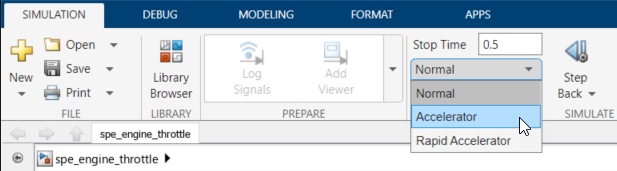Documentation

Use Accelerator Mode During Simulations

Simulink® Design Optimization™ software supports Normal and Accelerator simulation modes. You can accelerate the design optimization computations by changing the simulation mode of your Simulink model to Accelerator. For information about these modes, see How Acceleration Modes Work (Simulink).

The default simulation mode is Normal. In this mode, Simulink uses interpreted code, rather than compiled C code during simulations.

In the Accelerator mode, Simulink Design Optimization software runs simulations during optimization with compiled C code. Using compiled C code speeds up the simulations and reduces the time to optimize the model response signals.

Limitations

If the model structure changes during optimization, the model is compiled to regenerate the C code for each iteration. In this case, using the Accelerator mode increases the computation time. To learn more about code regeneration, see Code Regeneration in Accelerated Models (Simulink).

Setting Accelerator Mode

To set the simulation mode to Accelerator, open the Simulink model window and perform one of the following actions:

• Under Simulation, choose Accelerator from the drop-down list as shown in the next figure.Tip

To obtain the maximum performance from the Accelerator mode, close all Scope blocks in your model.

Related Topics

Simulink Design Optimization DocumentationGet trial now